# php基础教程 第四步 学习运算符

## +

echo $a+$b;


<?php
$a=9;$b=9;
echo $a+$b;
?>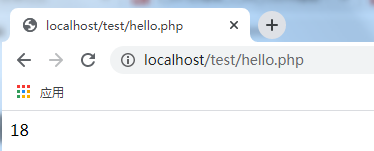# -

<?php
$a=9;$b=9;
echo $a-$b;
?>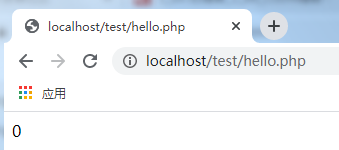# *

<?php
$a=9;$b=9;
echo $a*$b;
?>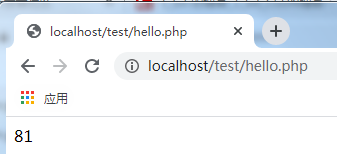# /

<?php
$a=9;$b=9;
echo $a/$b;
?>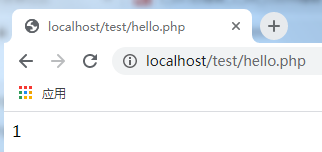# %

<?php
$a=9;$b=9;
echo $a%$b;
?>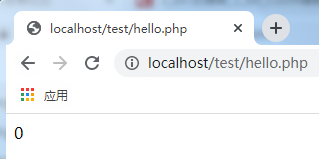## ==

echo a==b;


<?php
$a=9;$b=9;
echo $a==$b;
?>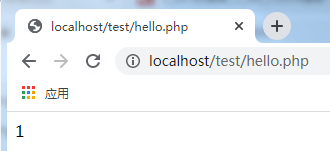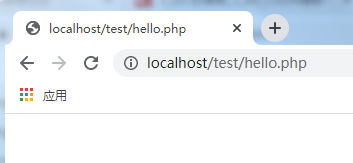## ++

<?php
$a=9; echo ++$a;
?>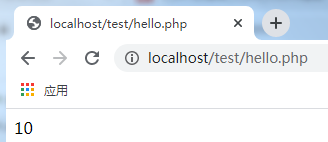++表示在当前变量的值基础上往上加一。

<?php
$a=9; echo$a++;
?>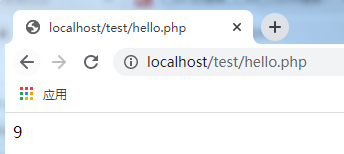echo $a++; echo '-'; echo$a;
?>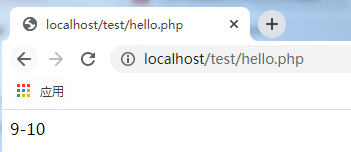# - -

- - 运算法与 + + 的使用方法类似，在当前变量值得基础上进行减1：

<?php
$a=9; echo$a--;
echo '-';
echo $a; ?>  运行结果如下：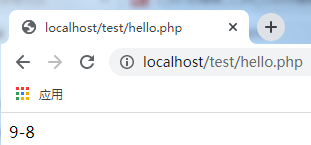- -放前面： <?php$a=9;
$b=10; echo$a!=$b; ?>  运行结果如下：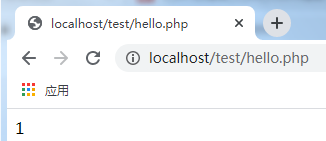因为在php中非0则表示判断正确。 把a和b的值改为一致，那么结果如下：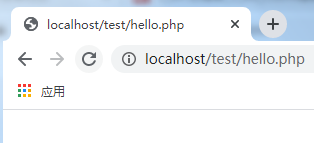## <、> 大于小于符号用来判断左右两边表达式或值是否判断正确。x>0判断x是否大于0，x>y判断x是否大于y。<号和>号用法一致，示例如下： <?php$a=10;
$b=9; echo$a>\$b;
?>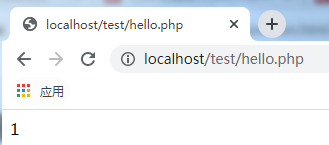## 其它运算法

php系列教程持续更细中，欢迎关注、点赞、收藏，谢谢支持。

08-0331207-131136
09-2026
11-24129
09-23538
06-24105
02-1850
07-2142